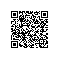# 一个可以把配置参数保存到数据库的函数

## 一个可以把配置参数保存到数据库的函数

/项目目录/Common/Common/function.php里就可以使用了。

function CD($key,$value = null){
/**
*    公共方法，可以把配置参数保存到数据库
*
*    用法：
*    CD('配置项');            //读取配置项
*    CD('配置项', '数据')    //写入配置项
*    CD('配置项', 'DEL')    //从数据库中移除该配置项
*
*
*在数据库中建立config表，表前缀改成自己的
* CREATE TABLE IF NOT EXISTS think_config (
*   id int(11) NOT NULL AUTO_INCREMENT,
*   key varchar(255) CHARACTER SET utf8 NOT NULL,
*   value varchar(255) CHARACTER SET utf8 NOT NULL,
*   PRIMARY KEY (id)
* ) ENGINE=MyISAM DEFAULT CHARSET=utf8 AUTO_INCREMENT=1 ;
*/
$cd = M('Config');$where = array( 'key' => $key); //如果有$value为空,就返回$key的值 if($value == null){
return $cd->where($where )->getField('value');
}
//如果$value为DEL,就删除这条记录的值 else if($value == "DEL")
{
return $cd->where($where )->delete();
}
//如果$value有正常值，其它操作 else{ //如果存在$key,更新
if($cd->where($where)->find()){
$cd->value =$value;
return $cd->where($where)->save();
}
//如果不存在$key，写入一条新数据 else{$cd->key = $key;$cd->value = $value; return$cd->add();
}
}
}使用钉钉扫一扫加入圈子
+ 订阅